# Introduction to the Theory of Cellular Automata and One-Dimensional Traffic Simulation

Richard Cochinos

## Abstract

This paper is roughly divided into two parts. After a brief introduction, I will discuss the theory and properties underlying cellular automata. Included in this section will be a definition, a list of the physical properties, rules and rules assigning, and a description of some of the dynamical properties inherent in automata. The second section of this paper will be devoted to discussing cellular automata as a model for traffic flow. It will include a one-dimensional example and conclusions from the model.

## Background

I became interested in cellular automata after engaging in a project to model human behavior. My idea for an art installation was to fill a gallery volume with twenty 8’ high columns, placed far enough apart so you could easily walk through them. The sides of the columns were to be painted various colors, with only one entrance/exit into the space. The question I was engaged with at the time was how would people react and interact with color when you present them with multiple distinct decisions. For the duration of the show I would record movement with a video camera placed on the ceiling. I was hoping to discover inherent patterns of movement across the floor, from various repellors to various attractors. I was then going to use what is known about color psychology to arrive at(if any) conclusions. The installation was never built due to the cost of the project and the time involved in construction. However, I decided to take the concept as far as I could intellectually. This led me to the problem of accurately describing the dynamics of people interacting with each other and objects within a finite space. I was then introduced to cellular automata as a modeling system. This paper is a continuation of that study.

## Introduction

The basis of my decision to examine cellular automata (CA) as a model for traffic was its logical parallelism to modeling human behavior through closed spaces. More and more metropolitan areas suffer from an increased transportation demand which largely exceeds capacity. In many cases it is not possible, or even desirable, to extend capacity to meet the demand. In consequence, the consistent management of large, distributed man-made transportation systems has become more and more important. However, until these are in place a reliable transportation system must exist. A first step in ‘reliability’ is understanding how these systems behave. CA’s are an alternative to Differential Equations in an attempt to model these systems. CA models have the desirable capacity to capture micro-level dynamics and relate these to macro-level behavior. Cellular Automata models are capable of representing individual vehicle interactions and relating these interactions to macroscopic traffic flow metrics, such as throughput, time travel and vehicle speed. By allowing different vehicles to possess different driving behaviors CA models can adequately capture the complexity of traffic.

This paper will mainly discuss the theory behind cellular automata. It is expansive, so not everything could be included. Before concluding I will work through a 1-dimentional traffic model as an example of several of the outlined concepts.

## Definition

Because of CA’s utility in so many disciplines, there are multiple ways in which an automaton can be defined. For our purposes I have concentrated on the physical and mathematical aspects which are necessary for understanding traffic simulation. The following are two universal definitions given by Lyman Hurd from Georgia Tech.:

Physicists View:
A cellular automaton is a discrete dynamical system. Each point in a regular spatial lattice, called a cell, can have any one of a finite number of states. The states in the cells of a lattice are updated according to a local rule. That is, the state of the cell at a given time depends only on its own state one time step previously, and the states of its nearby neighbors at the previous time step. All cells in the lattice are updated synchronously. The state of the lattice advances in discrete time steps.
Mathematicians View:
Notation: d=dimension
k=states per site
For simplicity, assume d=1 for the moment.
A d-dimensional cellular automaton takes as its underlying space the lattice (integers) where S is a finite set of k elements. The dynamics are determined by a global function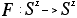whose dynamics are determined ``locally'' as defined below. A ``local (or neighborhood) function'' f is defined on a finite region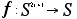The all-important property of cellular automata, is that this function is defined discretely (a finite lookup table). Both the domain and range of f are finite. The global function F arises from f by defining: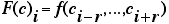(hurd@gatech.edu)

Some relevant facts from a topological standpoint are:
1. The base space is compact and the global function F is continuous.
2. The map F commutes with the shift operator which takes Ci to Ci+1.
In fact, cellular automata are characterized completely by properties 1 and 2 . According to Hurd, this makes CA are an ideal meeting point between continuous dynamics and complexity theory, since they are discretely defined but exhibit continuous dynamics. The transition to more dimensions is straightforward. The only difference is that the global function F is defined over S (functions from a d-dimensional lattice to S) and the local function f is determined by enumerating the image of all patches of size 2 (Gutowitz)

## Physical Properties

Because automata are not solely mathematics but modeling systems, it is helpful to understand the
physical properties of the automata. These can be divided into three parts, the cell and lattice, the neighborhood, and the rules.

The basic element of an automata is the cell. The cell is a memory element and stores states. In the simplest case each cell can have the binary states 1 or 0, or a finite amount of states in complex simulations. These cells are arranged in a spatial web -- a lattice. The simplest one being the one-dimensional lattice, meaning that all the cells are arranged in a line like a string of pearls. The most common CA’s are built in one or two dimensions, for computational limits. Because there is a much smaller possible set of 'rules', which will be discussed later, 1-D CA’s have been explored more by theoreticians.

Up to now these cells arranged in a lattice represent a static state. To introduce dynamic into the system, we have to add rules. The 'job' of these rules is to define the state of the cell for the next time in dependence of the neighborhood cells. Different definitions of neighborhoods are possible, each one being more or less effective depending on the system you wish to model. Concerning the two dimensional lattice the following definitions are common:

von Neumann Neighborhood
four cells, the cell above and below, right and left from each cell are called the von Neumann neighborhood of this cell. The radius of this definition is 1, as only the next layer is considered.
Moore Neighborhood
the Moore neighborhood is an enlargement of the von Neumann neighborhood containing the diagonal cells too. In this case, the radius r=1 too.
Extended Moore Neighborhood
equivalent to description of Moore neighborhood above, but neighborhood reaches over the distance of the next adjacent cells. Hence the r=2 (or larger).
Margolus Neighborhood
a completely different approach: considers 2x2 cells of a lattice at once.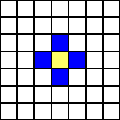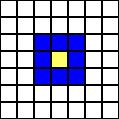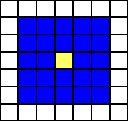von Neumann Neighborhood Moore Neighborhood Extended Moore Neighborhood

The blue cells are the neighborhood cells to the center (core) cell. As discussed before the states of these cells are used to calculate the next state of the center cell according to the defined rule. As the number the number of cells in a lattice has to be finite, one problem occurs considering the neighborhoods described above: What to do with cells at the borders? The influence depends on the size of the lattice. To give an example: In a 10x10 lattice about 40% of the cells are border cells, in a 100x100 lattice only about 4% of the cells are of that kind. Regardless, this problem must be solved. Two solutions are common:
1.Opposite borders of the lattice are "stuck together". A one-dimensional "line" becomes
a circle, a two dimensional lattice becomes a torus.
2. The border cells are mirrored: the consequence is symmetric border properties.
The more usual method chosen is (1) however, border definitions are infinite (Schatten).

## Rule Evolution of the CA

Evolutionary properties of the automata are properties that are affected by a rule. A nice example rule evolution is 'the wave' in a sports stadium. Each person reacts only to the state of his neighbor(s). If they stand up, (s)he will stand up too, and after a short while, (s)he sits down again. This illustrates the most important concept for automata: Local interaction leads to global dynamic. This dynamic is governed by rules. Interestingly all rules can be arranged into three classes:

1. Every group of states of the neighborhood cells is related a state of the core cell. E.g.
consider a one-dimensional CA: a rule could be "011 -> x0x", what means that the core cell
becomes a 0 in the next time step (generation) if the left cell is 0, the right cell is 1 and the core cell is 1. Every possible state has to be described.
2. "Totalistic" Rules: the state of the next state core cell is only dependent upon the sum of
the states of the neighborhood cells. E.g. if the sum of the adjacent cells is 4 the state of the
core cell is 1, in all other cases the state of the core cell is 0.
3. "Legal" Rules: a special kind of totalistic rules are the legal rules. As it is not of
advantage in most cases to use rules that produce a pattern from total zero-state lattices (all
cells in the automaton are 0), Wolfram defined the so called legal rules as a subset of all possible rules, a selection of rules that produce no ones from zero-state lattices (Schatten).

Rule 1 shows why one-dimensional automata are most popular in theoretical studies. If only the left, the right neighbor and the cell itself are considered(r=1), there are 256 possible rules. 2 =8 possible states for three binary digits. Each of these 8 states can produce a 1 or a 0 for the center cell in the next generation. Hence 2 =256 rules are existing. In general, the number of rules can be calculated by , where k is the number of states for the cell and n is the number of neighbors. We can easily see for a two-dimensional automata with a Moore neighborhood and 2 states, it follows k=2 and n=9. So =262,144 possible rules exist. A comprehensive study of all rules in higher dimensional automata is thus not easily possible (Schatten).

## Rule Assigning

Rules may be generalized in various ways. One family of rules is obtained by allowing the value of a site to be an arbitrary function of the values of the site itself and two of its nearest neighbors on the previous time step(rule class 1).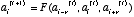It is possible to assign a convenient notation called ‘rule numbers’. Remember there are 256 rules of this type. Let us define the rule :

a(i)t+1=a(i-1)t+a(i+1)t mod 2 (1)

The figure below illustrates the conversion of all possible rule outcomes in binary code to hexadecimal code. Rule (1) is assigned the rule number 90 in this notation. As a note, rule numbers are not limited to functions of this type.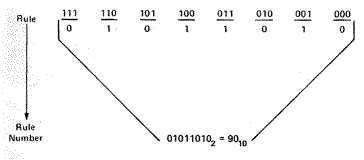As stated before, the number of different rules, with given k and n, grows by . The only problem is that for relatively small values of k and n the number of rules is immense. The figure below shows examples of evolution according to different rules with various k and r values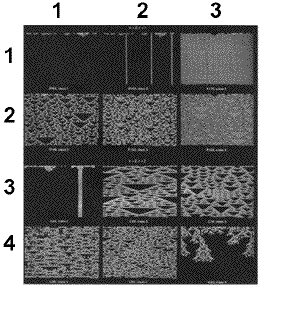(Wolfram 420)
Each separate rule leads to patterns that differ in detail, however the examples suggest a remarkable result: all patterns appear to fall into only four qualitative classes. Wolfram characterized these basic classes of behavior into:

• Class 1 – evolution tends to a homogeneous state (limiting points) in which, for example, all sites have value 0 – row 1 column 1, (1,1)
• Class 2 – evolution leads to a set of stable or periodic structures (limiting cycle), that are separate and simple - (1,2),(1,3),(3,1)
• Class 3 – evolution leads to a chaotic pattern or ‘strange attractor’– all remainder images except (4,3)
• Class 4 – evolution leads to complex structures, sometimes long-lived - image (4,3)

The existence of only 4 classes implies universality in the behavior of CA. The implications of this are large enough to extend beyond the scope of this paper. What is interesting though an adequate explanation has yet to be conjected.

## Properties of CA

Before moving on, I think it important to summarize the physical and evolutionary properties of cellular automata:

• CA´s develop in space and time
• a CA is a discrete simulation method, hence Space and Time are defined in discrete steps.
• a CA is built up from cells, that are lined up in a string for one-dimensional automata
arranged in a two or higher dimensional lattice for two- or higher dimensional automata
• the number of states of each cell is finite
• the states of each cell are discrete
• all cells are identical
• the future state of each cell depends only of the current state of the cell and the states of the cells in the neighborhood
• the development of each cell is defined by rules
• rules fall into three basic classes
• evolution of these rules leads to dynamical patterns that fall into four classes

## Dynamical properties

As CA simulations evolve ‘dynamical’ properties arise. Basically these are patterns we see again and again in varying models. Let us consider 1-D CA sites with the value of 0 or 1. Take the value of position i at time t to be a(i)t . For continuity we will use the same rule as before:

a(i)t+1=a(i-1)t+a(i+1)t mod 2 (1)

According to this rule, the value of a particular site is given by the sum modulo 2 of the values of
its left and right nearest neighbor sites on the previous time step, therefore, it is a totalistic rule.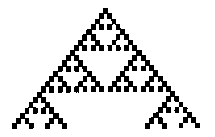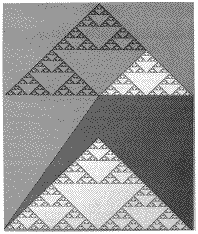figure 1figure 2

Figure 1 is an image of the evolution generated by eq (1) from a single seed after 23 time steps, figure 2 shows the pattern generated after 500 time steps. These are exemplifying 'self-similarity'. As illustrated, portions of the pattern when magnified are indistinguishable from the whole. Differences on a small scale between the original pattern and the magnified portion disappear when one considers the limiting pattern obtained after an infinite number of time steps. The pattern is therefore invariant under resealing of lengths. Such a self-similar pattern is often called a fractal and is characterized by a fractal diminution.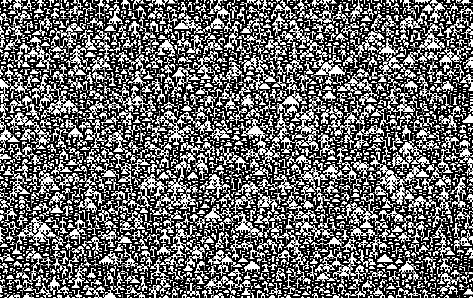The image above shows the evolution of equation 1 from a disordered initial state. The values of sites in the initial state are randomly chosen: each site taking on the value 0 or 1 with equal probability, independent of the values of other sites. Even though the initial state has no structure, evolution of the automata manifests some composition in the form of triangle ‘clearings’. The spontaneous appearance of these clearings is a simple example of ‘self-organization’. Both ‘self-similarity’ and ‘self-organization’ are well characterized collective phenomena, seen often in dynamical fields. The following two properties are intimately related governing how models are studied. These models are reversibility and irreversibility.

### Irreversibility

A notable feature in the image atop is that the trajectories merge with time. The merging of trajectories implies that information is lost in the evolution of the cellular automaton; the evolution is irreversible. The irreversible evolution decreases the possibility of some configurations and increases those for others. The possibility of self organization is therefore a consequence of irreversibility and the structures obtained through self-organization are determined by the characteristics of the attractors. This is an important concept in the fact that most natural systems exhibit irreversible trends in their evolution. CA models can mimic this behavior unlike statistical mechanics which exhibits reversible properties. While there is every evidence that the fundamental laws of physics are reversible, many systems behave irreversibly on a macroscopic scale and are appropriately described by irreversible laws. Cellular Automata provide mathematical models at this macroscopic level.

### Reversibility

The "only" difference to the CA´s described above is, that the time development of the system is completely reversible. That means, that at any time-step of the development, the rules allow to go forward or back in time without losing any information. Naturally most systems exhibit irreversible behavior, however constructed dynamical systems, such as traffic, usually counter this trend.

Now that we have briefly glazed over the important points in CA theory, we now move on to the construction and description of a concrete 1-D automaton traffic model.

## Traffic Flow models in literature

Traffic flow models can be divided into two major categories: microscopic and macroscopic. Microscopic models describe behavior as emerging from discrete entities interacting with each other. Macroscopic models are concerned with describing aggregate behavior by characterizing the fundamental relationships between vehicle speed, flow, and density (Benjaafar 3).
A major limitation of existing microscopic models is that they assume uniform behavior for all vehicles (ibid, 3). The models are deterministic, and therefore cannot capture inherent variation in real traffic vehicle behavior. Microscopic models are also difficult to extend to multi-lane systems. A key limitation of macroscopic simulation is their aggregate nature. Because they treat traffic flow as continuous, they are incapable of capturing the discrete dynamics which arise from the interactions of individual vehicles. For example, modeling different behaviors with regard to acceleration/deceleration or lane changing is difficult. In addition, because they are deterministic, these models can provide only average traffic flow metrics. Higher moments of throughput, travel time, and speed are impossible to characterize. The usefulness of most of these models is limited to characterizing the long run behavior of traffic flow and cannot be used for real time traffic analysis and control (ibid 3).

### Cellular Automata for one lane traffic flow

Our initial traffic model is defined as a one-dimensional array with L cells with closed (periodic) boundaries. This means the total number of vehicles N in the system is maintained constant. Each cell may be occupied by one vehicle or it may be empty. Each cell corresponds to a road segment with length l. Traffic density is the given by µ=N/L. Each velocity can have a velocity from 0 to vmax. The velocity corresponds to the number of sites that a vehicle advances in one iteration. The movement of vehicles throughout the cells is determined by a set of updating rules. These rules are applied in a parallel fashion to each vehicle at each iteration. The length of an iteration can be chosen to reflect the desired level of simulation detail. The choice of a sufficiently small iteration can thus be used to approximate a continuous time system. The state of a system at an iteration is determined by the distribution of vehicles among the cells and the speed of each vehicle in each cell. We adopt the following notation:

x(i): position of the ith vehicle
v(i): speed of the ith vehicle
g(i): gap between the ith and the (i+1)th vehicle, given by g(i)=x(i+1) - x(i) – 1

For our model we adapt the following set of rules:

1) Acceleration of free vehicles: If v(i)<vmax and g(i)>v(i)+1, then v(i+1)=v(i)+1
2) Slowing down due to other vehicles: If v(i)>g(i)-1, then v(i)=g(i)
3) Vehicle Motion: Vehicle is advanced v(i) sites

Figure 1 shows the application of these three updating rules to an example system with 24 cells and 7 vehicles: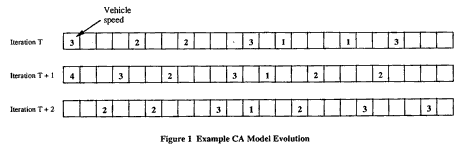Under these rules all vehicles have identical behavior and obey the same maximum speed, 5.
Benjaafar, Dooley and Setyawan, three graduate students at University of Minnesota, based a study on such a model. They were able to show that speed variance of the individual vehicles increased dramatically as the system approached maximum flow, the goal of most traffic plans. Often metro centers attempt to push roadways to maximum flow since they believe they are getting more cars through in the same finite amount of time. High speed variance means that different vehicles in the system have widely varying speeds. It also means that a vehicle would experience frequent speed changes per trip through the system. In turn, this results greater variability in the travel time. In reality, this increases the possibility of traffic accidents. Thus, it would seem reasonable to steer traffic away from the density of maximum flow, a recommendation counter to prevailing practice (Benjafarr 7).

Because we are using a deterministic, reversible and finite CA model with periodic boundaries, the corresponding traffic system is periodic in its system states. That is, the number of iterations necessary to bring back the same state is always finite. Again, Benjaafar et. al. were able to show that small changes in density could relate in large fluctuations in this period length. When applied to a ‘real’ situation, a car that immediately slows 5 mph during rush hour will have a significant impact on all those traveling behind it. This influence will take longer to dispense because of the density that time of day. In practice, a traffic system with a small period length is more desirable, being that system is easier to predict and control (Benjaafar 5). Although these examples are very intuitive more complex models show more complex behavior. It is not within the scope of this paper to discuss such systems.

Before closing I would like to inject that deterministic CA are useful as a modeling paradigm for automated highway systems, where vehicle speeding and vehicle deceleration are externally controlled. Indeed, an automated highway system would operate very much like a deterministic CA with rules specifying the total number of vehicles allowed in the system and the set of allowable vehicle behaviors.

## Conclusion

As stated before, CA’s developed as an alternative to partial differential equations. Like variations on counting systems, they are neither more or less effective, merely different. One of the nice feature cellular automata have is their information is not presented abstractly. Because all of the output of CA’s is in visual form, the average layman can understand the dynamics the model represents. In this paper I have attempted to give a brief overview of the theory behind automata as well as a concrete example in 1-D CA simulation. As stated in the background, this study began with the question of modeling human behavior in a finite closed space. From a programming standpoint, the transition from a one to a two-dimensional model is not that much more difficult. It is within the 2-D environment that the traffic modeling system becomes parallel with my original question. However promising this new system may seem, it should be stated the CA models are greatly simplified versions of reality. With advances in technology, newer computers are capable of more and faster calculations, hence greater accuracy. Like the notion of limits, these massively parallel machines are giving us insights into the fundamental behavior and pattern which surrounds our daily life, not through imitation – but approximation.

## Works Cited:

Richard Cochinos; email: richard@theory.org
Sat Jun 17 19:24:12 PDT 2000